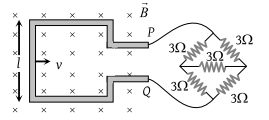# NEET Questions Solved

A square metallic wire loop of side 0.1 m and resistance of 1Ω is moved with a constant velocity in a magnetic field of 2 wb/m2 as shown in figure. The magnetic field is perpendicular to the plane of the loop, loop is connected to a network of resistances. What should be the velocity of loop so as to have a steady current of 1mA in loop(1) 1 cm/sec

(2) 2 cm/sec

(3) 3 cm/sec

(4) 4 cm/sec

Concept Videos :-

#6 | Motional EMF (Induced Electric Field)
#7 | Problem Set1: Motional EM
#8 | Problem Set2: Motional EM
#9 | Problem Set3: Motional EMF
#10 | Problem Set4: Motional EMF
#11 | Equivalent Circuit Diagrams

Concept Questions :-

Motional emf

(2) Equivalent resistance of the given Wheatstone bridge circuit (balanced) is 3Ω so total resistance in circuit is $R=3+1=4\Omega$. The emf induced in the loop e = Bvl.

So induced current $i=\frac{e}{R}=\frac{Bvl}{R}\text{\hspace{0.17em}\hspace{0.17em}\hspace{0.17em}}$

$⇒\text{\hspace{0.17em}\hspace{0.17em}\hspace{0.17em}}{10}^{-3}=\frac{2×v×\left(10×{10}^{-2}\right)}{4}⇒v=2cm/sec.$

Difficulty Level:

• 12%
• 68%
• 18%
• 4%
Crack NEET with Online Course - Free Trial (Offer Valid Till September 23, 2019)